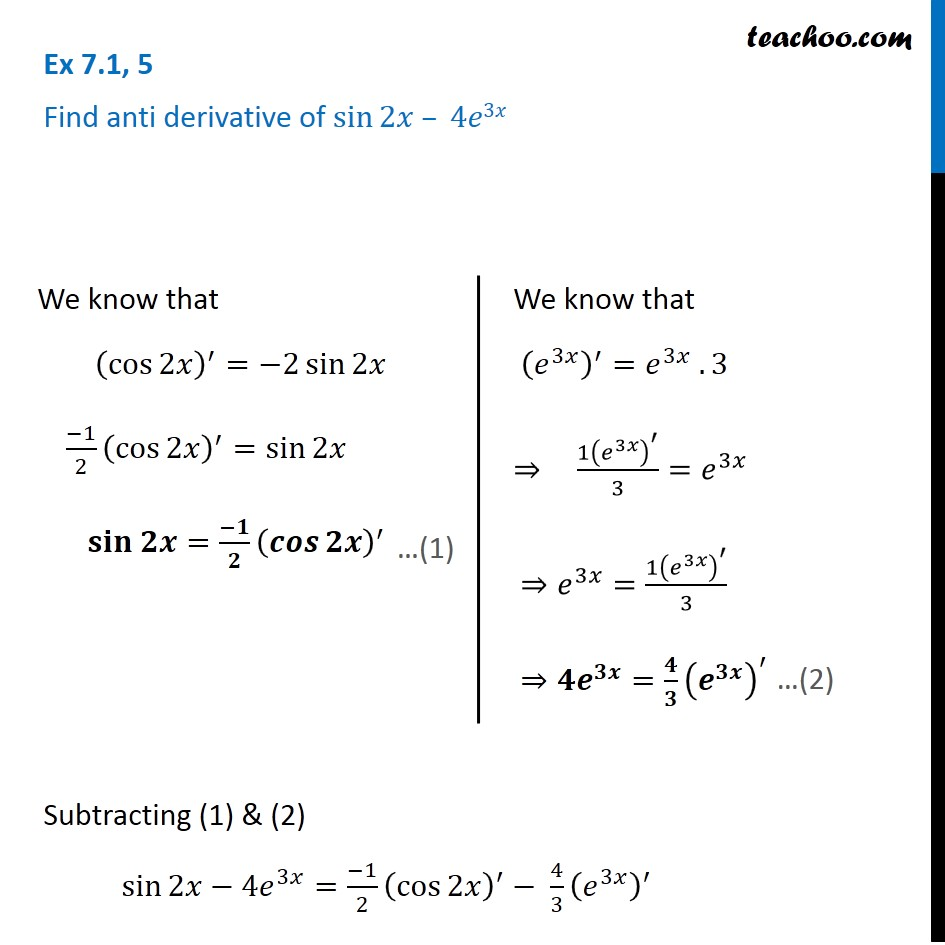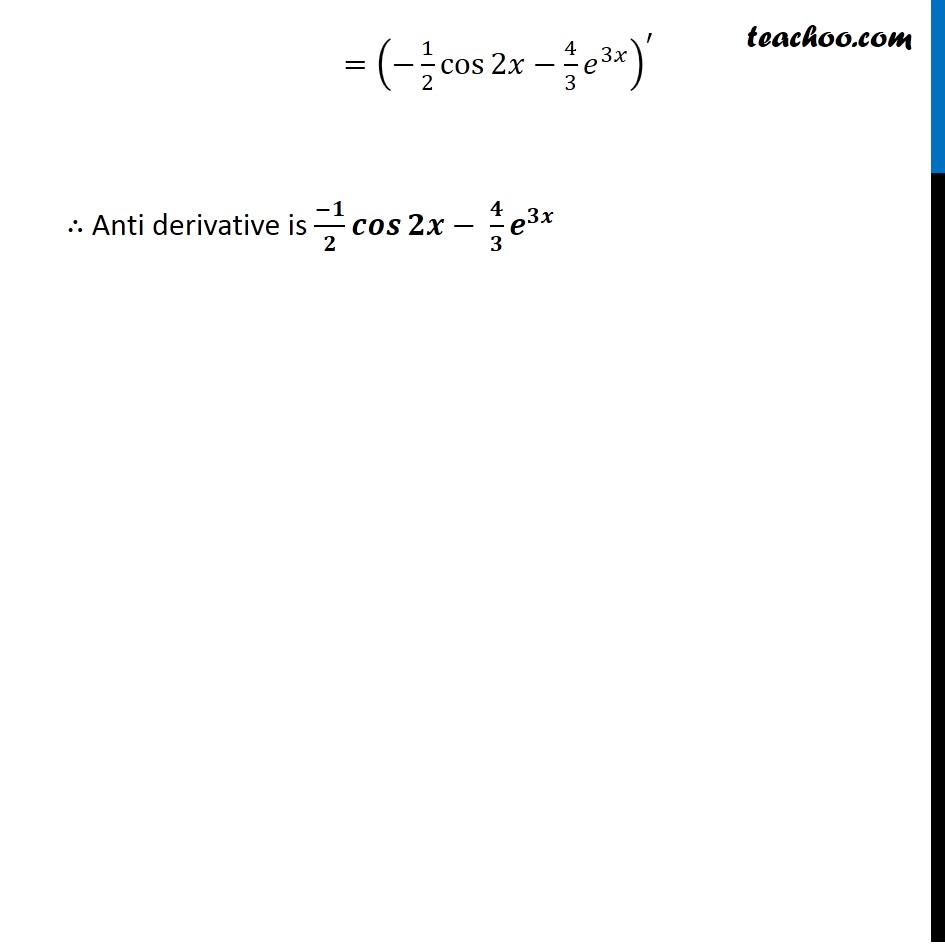Ex 7.1

Chapter 7 Class 12 Integrals
Serial order wiseLearn in your speed, with individual attention - Teachoo Maths 1-on-1 Class

### Transcript

Ex 7.1, 5 Find anti derivative of sin⁡2𝑥 – 4𝑒3𝑥 Subtracting (1) & (2) sin⁡2𝑥−〖4𝑒〗^3𝑥=(−1)/2 (cos⁡2𝑥 )^′− 4/3 (𝑒^3𝑥 )^′ We know that (cos⁡2𝑥 )^′=−2 sin⁡2𝑥 (−1)/2 (cos⁡2𝑥 )^′=sin⁡2𝑥 𝐬𝐢𝐧 𝟐𝒙=(−𝟏)/𝟐 (𝒄𝒐𝒔⁡𝟐𝒙 )^′ We know that (𝑒^3𝑥 )^′=𝑒^3𝑥 . 3 ⇒ 〖1(𝑒^3𝑥 )〗^′/3=𝑒^3𝑥 ⇒𝑒^3𝑥=〖1(𝑒^3𝑥 )〗^′/3 ⇒〖𝟒𝒆〗^𝟑𝒙=𝟒/𝟑 (𝒆^𝟑𝒙 )^′ …(2) =(−1/2 cos⁡2𝑥−4/3 𝑒^3𝑥 )^′ ∴ Anti derivative is (−𝟏)/𝟐 𝒄𝒐𝒔⁡𝟐𝒙− 𝟒/𝟑 𝒆^𝟑𝒙